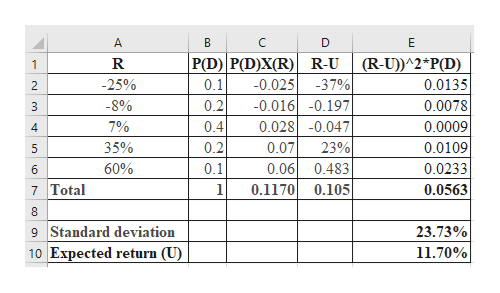# Expected Return: Discrete DistributionA stock's return has the following distribution:Demand for the Company's ProductsProbability of This Demand OccurringRate of Return if This Demand Occurs (%) Weak0.1-25% Below average0.2-8 Average0.47 Above average0.235 Strong0.160  1.0  Calculate the stock's expected return. Round your answer to two decimal places.___%Calculate the standard deviation. Round your answer to two decimal places.___%

Question
88 views

Expected Return: Discrete Distribution

A stock's return has the following distribution:

 Demand for the Company's Products Probability of This Demand Occurring Rate of Return if This Demand Occurs (%) Weak 0.1 -25% Below average 0.2 -8 Average 0.4 7 Above average 0.2 35 Strong 0.1 60 1.0

Calculate the stock's expected return. Round your answer to two decimal places.
___%

___%

check_circle

Step 1

Calculation of expected return and standard deviation:

The stock’s expected return is 11.70% and the standard deviation is 23.7...help_outlineImage TranscriptioncloseA B C P(D) P(D)X(R) R-U -37% -0.016-0.197 0.0280.047 (R-U))^2 P(D) 1 0.1 -25% 0.025 0.0135 2 -8% 0.2 0.0078 3 7% 0.4 0.0009 4 35% 0.2 0.07 23% 0.0109 5 0.06 0.483 0.1 60% 0.0233 6 0.0563 7 Total 0.1170 0.105 8 9 Standard deviation 10 Expected return (U) 23.73% 11.70% LC fullscreen

### Want to see the full answer?

See Solution

#### Want to see this answer and more?

Solutions are written by subject experts who are available 24/7. Questions are typically answered within 1 hour.*

See Solution
*Response times may vary by subject and question.
Tagged in